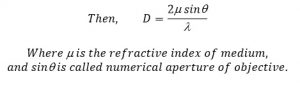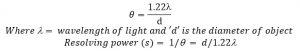# Diffraction Grating

An arrangement consisting of a large no of parallel slits. It is obtained by ruling the line on glass plate by diamond point. The line drawing on the plate aces as opaque and the space between two lines aces as transparent. Let ‘a’ be the width of opacities. Then (a + b) is known as grating element. If N be the no of lines drawn per unit length then
a + b = 1/N
Let a monochromatic light of wave length incidents on diffraction grating MN. Let the light wave are diffracted by N slits and converges by lens L on its focal plane i.e. at the screen XY ‘O’ be the position of central diffraction i.e. at the position of central maxima and ‘p’ be point at with light converges from the slits such that light wave are inclined by q to the initial direction . The path difference of light wave emitting from two consecutive slits and meeting at p is given by
Path difference = (a + b) sinq
For the secondary maxima at ‘p’
The path difference of light wave emitting from two consecutive slits should be integral multiple of l i.e.
(a+b) sinq = n, where n = 0,1,2,3…………. and n is order of diffraction.

Resolving power:
The ability of an instrument to produce separate distinct image of Hoo close object is called resolving power of instrument

Resolving power of instrument:
Consider a point object ‘o’ is illuminated by a light of wavelength and it is observed through the microscope.

If ‘o’ be the semi-vertical angle made by objective and ‘d’ be the least distance between two point object which can be distinguished.The resolving power is reciprocal of separation.

R.P=1/a = λ/2msinθ
The resolving power of telescope is reciprocal of smallest angular separation, between two distant object whose image are separated in telescope.
If θ be the angle subtended at object by objective then.